Berwald space

(diff) ← Older revision | Latest revision (diff) | Newer revision → (diff)

The Berwald connection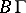satisfies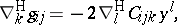wheredenotes the so-called Cartan torsion tensor. Clearly,if and only if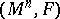is Riemannian (i.e.,has no dependence on). So, generally,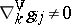nor is, generally,vanishing.

A Berwald spaceis a Finsler manifold (cf. Finsler space) such that its Berwald coefficientsare independent of. In fact,is a Berwald space if and only ifif and only if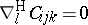.

Clearly, all Riemannian and locally Minkowski spaces are Berwald spaces (cf. also Riemannian space; Minkowski space). L. Berwald gave a complete characterization of such spaces. He used the frame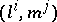and noted that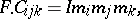whereis the so-called principal scalar invariant.

Berwald's theorem, slightly rephrased, reads as follows. Ifis a Berwald space which is not locally Minkowski (i.e.,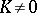), thenis a constant andhas one of the following four forms:

1),:2),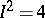: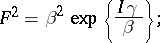3)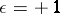,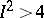: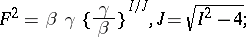4):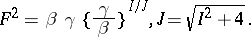Hereandare independent-forms in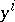that depend onand where the numberequalsifis positive definite and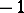otherwise (cf. also Berwald connection).

Applications of Berwald spaces in biology, physics and stochastic processes can be found in [a1], [a2].

How to Cite This Entry:
Berwald space. Encyclopedia of Mathematics. URL: http://encyclopediaofmath.org/index.php?title=Berwald_space&oldid=12731
This article was adapted from an original article by P.L. Antonelli (originator), which appeared in Encyclopedia of Mathematics - ISBN 1402006098. See original article